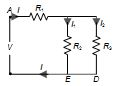## [LATEST]\$type=sticky\$show=home\$rm=0\$va=0\$count=4\$va=0

As per analysis for previous years, it has been observed that students preparing for NEET find Physics out of all the sections to be complex to handle and the majority of them are not able to comprehend the reason behind it. This problem arises especially because these aspirants appearing for the examination are more inclined to have a keen interest in Biology due to their medical background. Furthermore, sections such as Physics are dominantly based on theories, laws, numerical in comparison to a section of Biology which is more of fact-based, life sciences, and includes substantial explanations. By using the table given below, you easily and directly access to the topics and respective links of MCQs. Moreover, to make learning smooth and efficient, all the questions come with their supportive solutions to make utilization of time even more productive. Students will be covered for all their studies as the topics are available from basics to even the most advanced. .

Q1. 4 cells each of emf 2 V and internal resistance of 1Ω are connected in parallel to a load resistor of 2Ω. Then the current through the load resistor is?
•  2 A
•  1.5 A
•  1 A
•  0.888 A
Solution
I= E/(R+r/4)=2/(2+1/4)=2/2.25=0.888A

Q2. For ensuring dissipation of same energy in all three resistors (R1,R2,R3)connected as shown in figure, their values be related as?
•  R1=R2=R3
•  R2=R3 and R1=4 R2
•  R2=R3 and R1 =R2/4
•  R1= R2+R3
Solution
As voltage across the resistors R2 and R3 is same and they show same dissipation of energy, so using the relation for energy, H=V2/R t,we have R2=R3. Thus, the current in each resistor R2 and R3 will be I/2 ie,I1=I/2 and I2=I/2Since the energy dissipation is same in all the three resistors, so I2 R1 t=I12 R2 t or I2 R1 t=(I/2)2 R2 t or R1=R2/4

Q3. n identical cells, each of emf E and internal resistance r, are connected in series a cell A is joined with reverse polarity. The potential difference across each cell, except A is?
•  2nE/(n-2)
•  (n-2)E/n
•  (n-1)E/n
•  2E/n
Solution
When one call is wrongly connected in series, the emf of cells decrease by 2 E, but internal resistance of cells remains the same for all the cells. Current in the circuit is i=(n-2)E/nr×r Potential difference across each cell is V=E-Ir=E-(n-2)E/nr×r=2E/n

Q4. A current of A enters one corner one corner P of an equilateral triangle PQR having 3 wires of resistance 2 Ω each and leaves by the corner R. then the current I1 and I2 are?
•  2 A, 4 A
•  4 A, 2 A
•  1 A, 2 A
•  2 A, 3 A
Solution
From Kirchhoff’s first law at junction P, I1+I2=6 …(i) From Kirchhoff’s second law to the closed circuit PQRP, -2I1-2I1+2I2=0 -4I1+2I2=0 2I1-I2=0 ……(ii) Adding Eqs. (i) and (ii), we get 3I1=6 I1=2A From Eq. (i), I2=6-2=4A

Q5. 160W-60V lamp is connected at 60 V DC supply. The number of electrons passing through the lamp in 1 min is (the charge of electron e=1.6×10-19 C)?
•  4.5 ohm1019
•  1021
•  1.6×1019
•  1.4×1020
Solution
Power, P=V2/R R=V2/P=(60)2/160=22.5Ω Now, according to Ohm’s law V=IR ∴ I=60/22.5 I=2.6A Here, t=60s As I=ne/t n=(I×t)/e = (26×60)/(1.6×10-19 )≈1021

Q6. If a 30 V,90 W bulb is to be worked on a 120 V line, a resistance of how many ohms should be connected in series with the bulb?
•  10 ohm
•  20 ohm
•  30 ohm
•  40 ohm
Solution
Suppose resistance R is corrected in series with bulb Current through the bulb i=90/30=3 AHence for resistance V=iR⇒90=3×R⇒R=30Ω

Q7. In a thermo-couple, one junction which is at 0℃ and the othe at t℃ the emf is given by E=at2-bt2. The neutral temperature is given by?
•  a/b
•  2 a/3b
•  3a/2b
•  b/2a
Solution
dT/dt=d/dt (at2-bt3 )=2at-3bt2 When t=tn (ie, neutral temperature), dE/dt=0 ∴0=2atn-3btn2 ortn=2a/3b.

Q8. 5 cells, each of emf 0.2 V and internal resistance 1 Ω are connected to an external circuit of resistance of 10 Ω. Find the current through external circuit?
•  1/2.5 A
•  1/10 A
•  1/15 A
•  1/2 A
Solution

Here, emf of each cell, ε=0.2V Internal resistance of each cell, r=1Ω External resistance, R=10Ω The total emf of 5 cells =5ε=5(0.2)V=1V Total internal resistance of 5 cells =5r=5(1)Ω=5Ω Total resistance of the circuit =R+5r=10+5=15Ω The current in the external circuit, I=5ε/(R+5r)=1V/15Ω=1/15 A

Q9. Two heater wires of equal length are first connected in series and then in parallel. The ratio of heat produced in the two cases is?
•  1 : 4
•  4 : 1
•  1 : 2
•  2 : 1
Solution
Let the resistance of each heater wire is R. When two wires are connected in series, the heat developed is H1=(V2 t)/2R …(i) When two heater wires are connected in parallel, the heat developed is H2=(V2 t)/(R/2)=(2V2 t)/R …(ii) Dividing Eq. (i) by Eq. (ii), we get H1/H2 =1/4 or H1 ∶H2=1∶4 .

Q10. A combination of two resistance of 2 W and 2/3 W connected in parallel is joined across a battery of emf of 3 V and of negligible internal resistance. The energy given out per sec will be?
•  1/2×3×3 J
•  1/2×1/3×3×3
•  2×3 J
•  3×3×2 J
Solution
Total power spend across two resistors connected in parallel to battery =V2/R1 +V2/R2 =(3×3)/2+(3×3)/(2/3)=36/2=18 =3×3×2 J## Want to know more

Please fill in the details below:

## Latest NEET Articles\$type=three\$c=3\$author=hide\$comment=hide\$rm=hide\$date=hide\$snippet=hide

Name

ltr
item
BEST NEET COACHING CENTER | BEST IIT JEE COACHING INSTITUTE | BEST NEET & IIT JEE COACHING: Current Electricity Quiz 14
Current Electricity Quiz 14